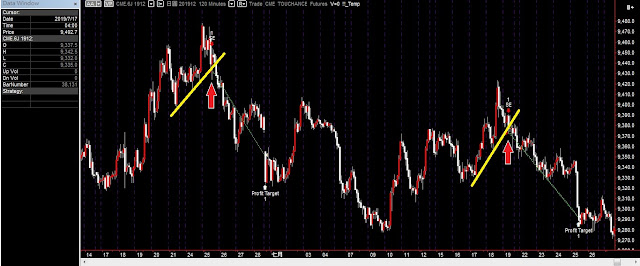## 2019年11月4日 星期一

### ★趨勢線指標及策略(含程式碼)

當我們在用撰寫程式交易策略的時候，為了方便，經常會使用近期的高低點作為突破型策略的進場點，但隨著程式交易的人口增加突破近期高低點才追價進場太慢了，而且很容易滑價。所以今天跟大家分享一個趨勢線指標，簡單來說就是藉由近期高點或低點的連線，來作為突破點，就跟老派的分析師一樣，在K線圖上畫線，當出現突破的時候，就直接追價。只是要把這種趨勢線寫進程式交易並不容易，也因為這個原因，寫出來的策略進場點常常可以跟大家不一樣，用來分散突破型策略的滑價風險也是一個選擇。日圓期貨使用趨勢線突破進場的示意圖

要畫趨勢線最簡單的方法就是拿起一支筆，在K棒上把波峰的高點相連，或近期波谷的低點相連，延伸出去的那條就是趨勢線了，這種型態的指標用手畫很簡單，但是要把它寫成程式碼就很頭痛了，為了要方便在程式中使用，我把趨勢線寫成了函數，提供給讀者學習。然而趨勢線的畫法很多，在國外論壇可以買更精美的程式語法，只是不見得合自己胃口。

=================================================

★趨勢線函數程式 TL_Function的程式碼如下： (把下面這段寫在函數中)

input:PriceValue(NumericSeries),
Length(NumericSimple),
UpOrDn(TrueFalse),
ShowTrendLine(TrueFalse),
SetColor(NumericSimple);

var:MA(0),Tri(0),Slope(0),
Tri1Bar(0),Tri3Bar(0),
Tri1Date(0),Tri3Date(0),
Tri1Time(0),Tri3Time(0),
Tri1Price(0),Tri3Price(0),flag(0);
//var:NodPt(0);
/////=====Up Triangle=======/////
if UpOrDn=true then begin
MA=highest(PriceValue,Length);
flag=0;
switch Tri begin
case 0:
if MA<MA then begin
Tri=1;
Tri1Bar=currentbar-Length;
Tri1Price=PriceValue[Length];
Tri1Date=d[Length];
Tri1Time=t[Length];
end;
case 1:
if MA>MA then begin
Tri=2;
end;
case 2:
if MA<MA then begin
flag=1;
Tri=1;
Tri3Bar=currentbar-Length;
Tri3Price=PriceValue[Length];
Tri3Date=d[Length];
Tri3Time=t[Length];
Slope=iff(Tri3Bar-Tri1Bar=0,0,(Tri3Price-Tri1Price)/(Tri3Bar-Tri1Bar));
if ShowTrendLine=true then begin
value1=tl_new(Tri1Date,Tri1Time,Tri1Price,Tri3Date,Tri3Time,Tri3Price);
tl_setcolor(value1,SetColor);
end;
Tri1Bar=currentbar-Length;
Tri1Price=PriceValue[Length];
Tri1Date=d[Length];
Tri1Time=t[Length];
//             NodPt=Tri1Price;
//             text_new(Tri1Date,Tri1Time,Tri1Price+20,NumToStr(NodPt,0));
end;
end;
end;

/////============Dn Triangle============/////
if UpOrDn=False then begin
MA=lowest(PriceValue,Length);
flag=0;
switch Tri begin
case 0:
if MA>MA then begin
Tri=1;
Tri1Bar=currentbar-Length;
Tri1Price=PriceValue[Length];
Tri1Date=d[Length];
Tri1Time=t[Length];
end;
case 1:
if MA<MA then begin
Tri=2;
end;
case 2:
if MA>MA then begin
flag=1;
Tri=1;
Tri3Bar=currentbar-Length;
Tri3Price=PriceValue[Length];
Tri3Date=d[Length];
Tri3Time=t[Length];
Slope=iff(Tri3Bar-Tri1Bar=0,0,(Tri3Price-Tri1Price)/(Tri3Bar-Tri1Bar));
if ShowTrendLine=true then begin
value2=tl_new(Tri1Date,Tri1Time,Tri1Price,Tri3Date,Tri3Time,Tri3Price);
tl_setcolor(value2,SetColor);
end;
Tri1Bar=currentbar-Length;
Tri1Price=PriceValue[Length];
Tri1Date=d[Length];
Tri1Time=t[Length];
//             NodPt=Tri1Price;
//             text_new(Tri1Date,Tri1Time,Tri1Price-20,NumToStr(NodPt,0));
end;
end;
end;

Function_TL=(currentbar-Tri1Bar)*Slope+Tri1Price;
if ShowTrendLine=true then begin
if flag=flag then begin
value3=tl_new(d,t,Function_TL,d,t,Function_TL);
tl_setcolor(value3,setcolor);
end;
if Tri=2 and Tri=1 then begin
value4=tl_new(Tri3Date,Tri3Time,Tri3Price,d,t,Function_TL);
tl_setcolor(value4,setcolor);
end;
end;

=================================================

(1)   PriceValue是要取峰谷值的value，通常壓力線在上方，所以會取high，支撐線在下方，所以會取low。當然也可以將成交量或是RSI這些指標餵給Function_TL運算。
(2)   Length用來調整趨勢線的敏感度，也就是峰值highest(h,len)或谷值lowest(l,len)裡面的那個len
(3)   UpOrDn是用來選擇我們是要峰峰相連還是谷谷相連，像壓力線是峰峰相連，就用true，支撐線是谷谷相連就用false
(4)   ShowTrendLine這個只是用來控制要不要把相連的壓力線和支撐線畫在圖表上，一般交易的時候都用false關掉，以減少資源的耗用。
(5)   SetColor是設定畫出來的線的顏色，當然如果ShowTrenLinefalse的話，這個參數就沒有用了，隨便設一個就可以。

●CME的日圓期貨，回測如下：

●台指期(TXF)回測如下：

K棒週期：120分K (08:45~13:45)

#### 張貼留言

----------------------------------------------------------------------------------------------------

-------------------------------------------------------------------------------------------------